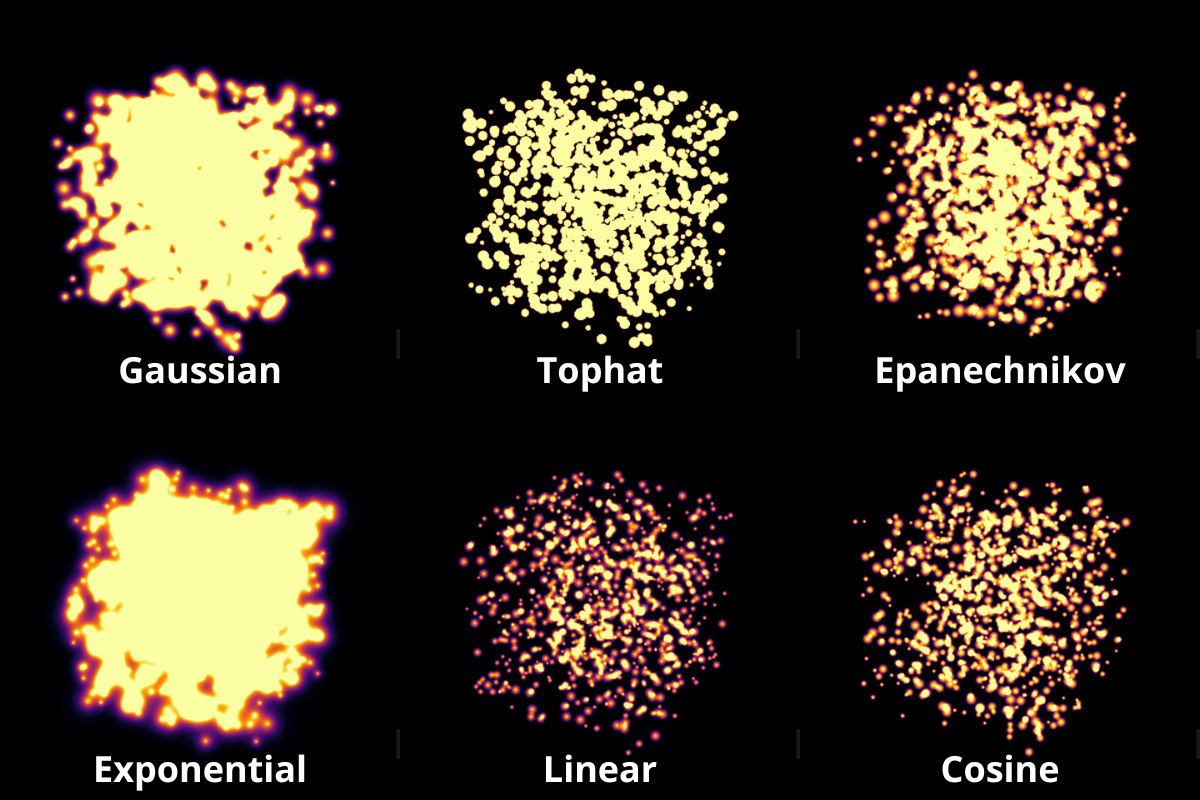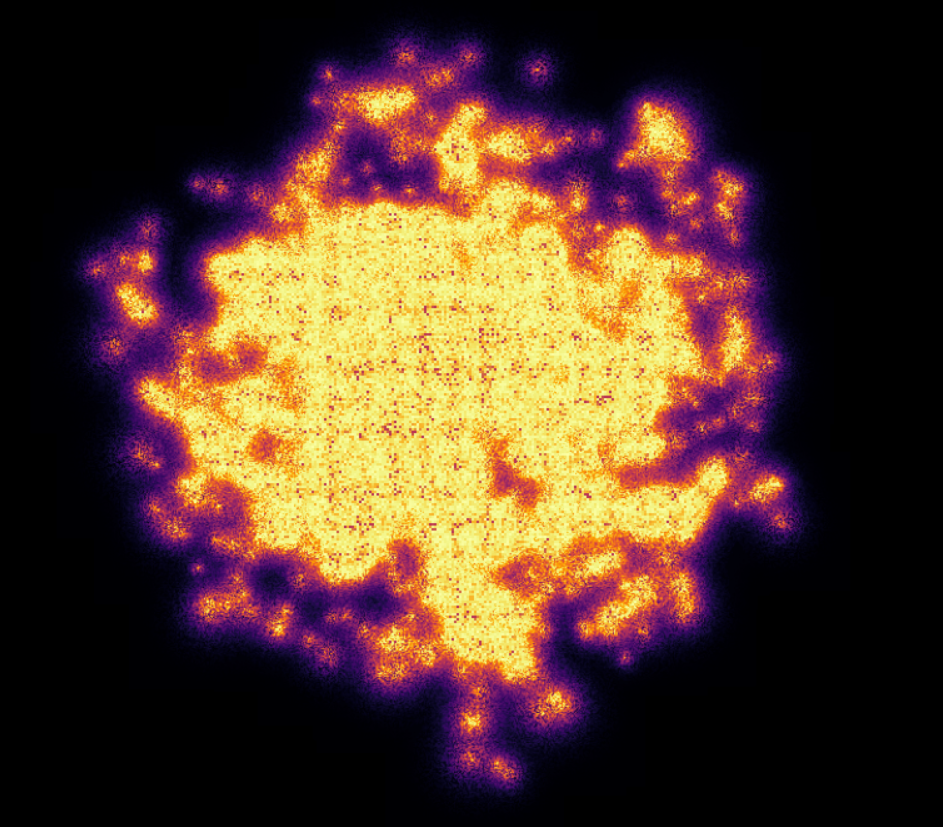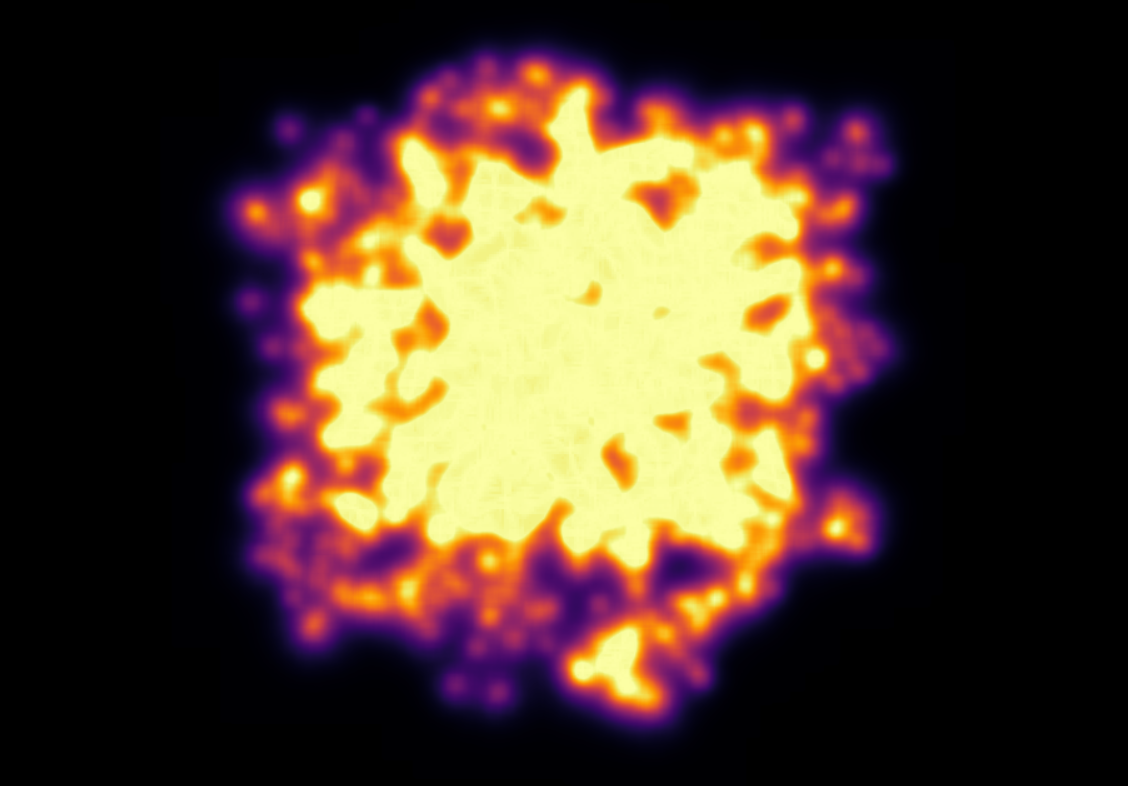# Week 9: It is Polishing Time!#

Hello everyone, it’s time for another weekly blogpost! Today, I am going to update you on my project’s latest changes.

## Last Week’s Effort#

After having finished a first draft of the API that will be used for the KDE rendering, and showing how it could be used for other post-processing effects, my goal was to clean the code and try some details that would add to it so it could be better complete. Having that in mind, I invested in three work fronts:

1. Fixing some bugs related to the rendering more than one post-processing effect actor.

2. Experimenting with other rendering kernels (I was using the gaussian one only).

3. Completing the KDE render by renormalizing the values in relation to the number of points (one of the core KDE details).

Both three turned out more complicated than it initially seemed, as I will show below.

## So how did it go?#

The first one I did on monday-tuesday, and I had to deal with some issues regarding scaling and repositioning. Due to implementation choices, the final post-processed effects were rendered either bigger than they were in reality, or out of their original place. After some time dedicated to finding the root of the problems, I could fix the scaling issue, however I realised I would need to, probably, rethink the way the API was implemented. As this general post-processing effects is a side-project that comes as a consequence of my main one, I decided to leave that investment to another time, as I would need to guarantee the quality of the second.

The second was an easy and rather interesting part of my week, as I just needed to setup new kernel shaders. Based on scikit-learn KDE documentation, I could successfully implement the following kernels:

• Gaussian

$K(x, y) = e^{\frac{-(x^2 + y^2)}{2\sigma^2}}$
• Tophat

$K(x, y) = 1.0, \ \ |x^2 + y^2| < \sigma$
• Epanechnikov

$K(x, y) = 1 - \frac{x^2 + y^2}{\sigma^2}$
• Exponential

$K(x, y) = e^{\frac{-|x^2 + y^2|}{\sigma}}$
• Linear

$K(x, y) = 1 - \frac{|x^2 + y^2|}{\sigma}, \ \ |x^2 + y^2| < \sigma$
• Cosine

$K(x, y) = cos(\frac{\pi|x^2 + y^2|}{2\sigma})$

That outputted the following (beautiful) results for a set of 1000 random points with random sigmas:The third one is still being a trickier challenge. If you recall from my first blogposts, I spent something around one month trying to setup float framebuffer objects to FURY with VTK so I could use them in my project. After spending all of that time with no results, me and Bruno, my mentor, found a way to do what we wanted to do, but using a different VTK class, vtkWindowToImageFilter. Well, it was a good workaround back then and it lead me all the way here, however now it is costing a price. The float framebuffers were an important part of the project because they would allow us to pass 32-bit float information from one shader to another, which would be important as they would allow the densities to have higher precision and more fidelity to the calculations. When rendering a KDE of a given set of points, we use the below function:

$KDE(x, y) = \frac{1}{n} \sum_{i = 0}^n K(x, y)$

If the number of points $$n$$ is big enough, some KDE results will be really low. This presents a real problem to our implementation because, without the float framebuffers, it is currently only being possible to pass 8-bit unsigned char information, that only allows 256 values. This is far from ideal, as low values would have alone densities low enough to disappear. This presented a problem as to renormalize the densities, I was retrieving the texture to the CPU, calculating its minimum and maximum values, and passing to the fragment shader as uniforms for the renormalization, which didn’t work if the maximum values calculated were zero.

One solution I thought to solve that was a really heavy workaround: if an unsigned float is 32-bit and I have exactly 4 8-bit unsigned chars, why not try to pack this float into these 4 chars? Well, this is an interesting approach which I figured out is already an old one, being reported in GPU Gems’s chapter 12. Unfortunately I haven’t tried yet this implementation yet, and went for one I thought myself, which haven’t exactly worked. I also tried this implementation from Aras Pranckevičius’ website, which seems to be working, even though not perfectly:As you can see, this implementation is really noisy. I think this has to deal with floating point rounding errors, so to try to mitigate that, I experimented applying a 13x13 gaussian blur to it. Below, what I got from that:That looks way better, even though not ideal yet.

## This Week’s Goals#

Talking with my mentors, we decided it was better if I focused on the version without the renormalization for now, as it was already done and running fine. So for this week, I plan to clean my PR to finally have it ready for a first review, and maybe add to it a little UI tool to control the intensity of the densities. That should take me some time and discussion, but I hope for it to be ready by the end of the week.

Let’s get to work!